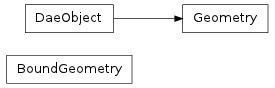#### Next topic

A class containing the data coming from a COLLADA <geometry> tag__init__(collada, id, name, sourcebyid, primitives=None, xmlnode=None, double_sided=False)

Create a geometry instance

Parameters: collada (collada.Collada) – The collada object this geometry belongs to id (str) – A unique string identifier for the geometry name (str) – A text string naming the geometry sourcebyid – A list of collada.source.Source objects or a dictionary mapping source ids to the actual objects primitives (list) – List of primitive objects contained within the geometry. Do not set this argument manually. Instead, create a collada.geometry.Geometry first and then append to primitives with the create* functions. xmlnode – When loaded, the xmlnode it comes from. double_sided (bool) – Whether or not the geometry should be rendered double sided

Methods

 __init__(collada, id, name, sourcebyid[, ...]) Create a geometry instance bind(matrix, materialnodebysymbol) Binds this geometry to a transform matrix and material mapping. createLineSet(indices, inputlist, materialid) Create a set of lines for use in this geometry instance. createPolylist(indices, vcounts, inputlist, ...) Create a polylist for use with this geometry instance. createPolygons(indices, inputlist, materialid) Create a polygons for use with this geometry instance. createTriangleSet(indices, inputlist, materialid) Create a set of triangles for use in this geometry instance. load(collada, localscope, node) save() Saves the geometry back to xmlnode

id

The unique string identifier for the geometry

name

The text string naming the geometry

double_sided

A boolean indicating whether or not the geometry should be rendered double sided

sourceById

A dictionary containing collada.source.Source objects indexed by their id.

primitives

List of primitives (base type collada.primitive.Primitive) inside this geometry.

xmlnode

ElementTree representation of the geometry.

createLineSet(indices, inputlist, materialid)

Create a set of lines for use in this geometry instance.

Parameters: indices (numpy.array) – unshaped numpy array that contains the indices for the inputs referenced in inputlist inputlist (collada.source.InputList) – The inputs for this primitive materialid (str) – A string containing a symbol that will get used to bind this lineset to a material when instantiating into a scene collada.lineset.LineSet
createTriangleSet(indices, inputlist, materialid)

Create a set of triangles for use in this geometry instance.

Parameters: indices (numpy.array) – unshaped numpy array that contains the indices for the inputs referenced in inputlist inputlist (collada.source.InputList) – The inputs for this primitive materialid (str) – A string containing a symbol that will get used to bind this triangleset to a material when instantiating into a scene collada.triangleset.TriangleSet
createPolylist(indices, vcounts, inputlist, materialid)

Create a polylist for use with this geometry instance.

Parameters: indices (numpy.array) – unshaped numpy array that contains the indices for the inputs referenced in inputlist vcounts (numpy.array) – unshaped numpy array that contains the vertex count for each polygon in this polylist inputlist (collada.source.InputList) – The inputs for this primitive materialid (str) – A string containing a symbol that will get used to bind this polylist to a material when instantiating into a scene collada.polylist.Polylist
createPolygons(indices, inputlist, materialid)

Create a polygons for use with this geometry instance.

Parameters: indices (numpy.array) – list of unshaped numpy arrays that each contain the indices for a single polygon inputlist (collada.source.InputList) – The inputs for this primitive materialid (str) – A string containing a symbol that will get used to bind this polygons to a material when instantiating into a scene collada.polygons.Polygons
save()

Saves the geometry back to xmlnode

bind(matrix, materialnodebysymbol)

Binds this geometry to a transform matrix and material mapping. The geometry’s points get transformed by the given matrix and its inputs get mapped to the given materials.

Parameters: matrix (numpy.array) – A 4x4 numpy float matrix materialnodebysymbol (dict) – A dictionary with the material symbols inside the primitive assigned to collada.scene.MaterialNode defined in the scene collada.geometry.BoundGeometry